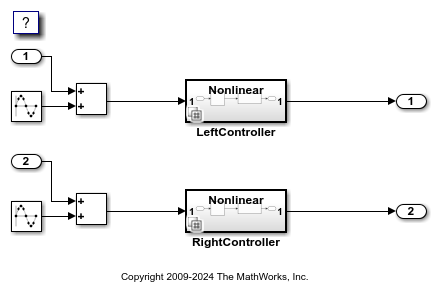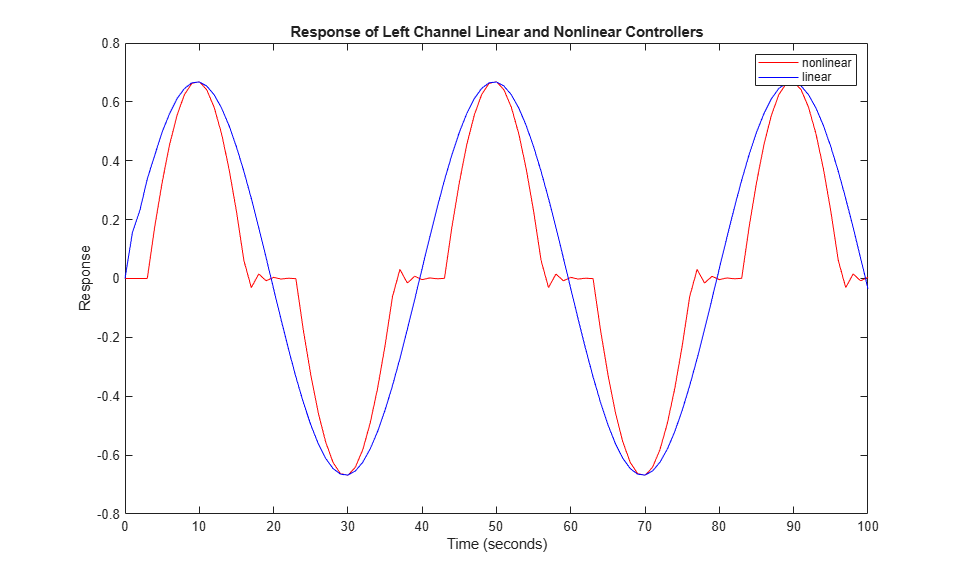# Use Variant Subsystem To Generate Code That Uses C Preprocessor Conditionals

This example shows how to use Simulink® variant subsystems to generate C preprocessor conditionals that control which child subsystem of the variant subsystem is active in the generated code produced by the Simulink® Coder™.

### Overview of Variant Subsystems

A Variant Subsystem block contains two or more child subsystems where one child is active during model execution. The active child subsystem is referred to as the active variant. You can programmatically switch the active variant of the Variant Subsystem block by changing values of variables in the base workspace, or by manually overriding variant selection using the Variant Subsystem block dialog. The active variant is programmatically wired to the Inport and Outport blocks of the Variant Subsystem by Simulink® during model compilation.

To programmatically control variant selection, a `Simulink.Variant` object is associated with each child subsystem in the Variant Subsystem block dialog. `Simulink.Variant` objects are created in the MATLAB® base workspace. These objects have a property named `Condition`, an expression, which evaluates to a Boolean value and is used to determine the active variant child subsystem.

By default the generated code contains only the active variant. Alternatively, you can parameterize the selection of the active variant, and make it dependent on the values of variables and objects in the base MATLAB® workspace. When you generate code, you can generate code for all variants and defer the choice of active variant until it is time to compile that code.

### Specifying Variants for a Subsystem Block

Opening the example model `rtwdemo_preprocessor_subsys` will run the PostLoadFcn defined in the "File: ModelProperties: Callbacks" dialog. This will populate the base workspace with the variables for the Variant Subsystem blocks.

```open_system('rtwdemo_preprocessor_subsys') ```The LeftController variant subsystem contains two child subsystems: Linear and Nonlinear. The LeftController/Linear child subsystem executes when the Simulink.Variant object LINEAR evaluates to `true`, and the LeftController/Nonlinear child subsystem executes when the Simulink.Variant object NONLINEAR evaluates to `true`.

Simulink.Variant objects are specified for the LeftController subsystem by right-clicking the LeftController subsystem and selecting `Subsystem Parameters`, which will open the LeftController subsystem block dialog.

```open_system('rtwdemo_preprocessor_subsys/LeftController'); ```The LeftController subsystem block dialog creates an association between the Linear and Nonlinear subsystems with the two Simulink.Variant objects, `LINEAR` and `NONLINEAR`, that exist in the base workspace. These objects have a property named `Condition`, an expression, which evaluates to a Boolean value and determines the active variant child subsystem (Linear or Nonlinear). The condition is also shown in the subsystem block dialog. In this example, the conditions of `LINEAR` and `NONLINEAR` are 'VSSMODE == 0' and 'VSSMODE == 1', respectively.

In this example, the Simulink.Variant objects are created in the base workspace.

```LINEAR = Simulink.Variant; LINEAR.Condition = 'VSSMODE==0'; NONLINEAR = Simulink.Variant; NONLINEAR.Condition = 'VSSMODE==1'; ```

### Specifying a Variant Control Variable

Variant objects allow you to reuse arbitrarily complex conditions throughout a model. Multiple Variant Subsystem blocks can use the same Simulink.Variant objects, allowing you to toggle the activation of choices as a set. You can toggle the set prior to simulation by changing the value of `VSSMODE` in the MATLAB environment or when compiling the generated code, as explained in the next section. In this example, LeftController and RightController reference the same variant objects, so that you can toggle them simultaneously.

The nonlinear controller subsystems implement hysteresis, while the linear controller subsystems act as simple low-pass filters. Open the subsystem for the left channel. The subsystems for the right channel are similar.

The generated code accesses the variant control variable `VSSMODE` as a user-defined macro. In this example, `rtwdemo_importedmacros.h` supplies `VSSMODE`. Within the MATLAB environment, you specify `VSSMODE` using a `Simulink.Parameter` object. Its value will be ignored when generating code including preprocessor conditionals. However, the value is used for simulation. The legacy header file specifies the value of the macro to be used when compiling the generated code, which ultimately activates one of the two specified variants in the embedded executable.

Variant control variables can be defined as `Simulink.Parameter` objects with one of these storage classes:

• `Define` or `ImportedDefine` with header file specified

• `CompilerFlag`

• `SystemConstant (AUTOSAR)`

• User-defined custom storage class that defines data as a macro in a specified header file

```VSSMODE = Simulink.Parameter; VSSMODE.Value = 1; VSSMODE.DataType = 'int32'; VSSMODE.CoderInfo.StorageClass = 'Custom'; VSSMODE.CoderInfo.CustomStorageClass = 'ImportedDefine'; VSSMODE.CoderInfo.CustomAttributes.HeaderFile = 'rtwdemo_importedmacros.h'; ```

### Simulating the Model with Different Variants

Because you set the value of `VSSMODE` to 1, the model uses the nonlinear controllers during simulation.

```sim('rtwdemo_preprocessor_subsys') youtnl = yout; ```

If you change the value of `VSSMODE` to 0, the model uses the linear controllers during simulation.

```VSSMODE.Value = int32(0); sim('rtwdemo_preprocessor_subsys') youtl = yout; ```

You can plot and compare the response of the linear and nonlinear controllers:

```figure('Tag','CloseMe'); plot(tout, youtnl.signals(1).values, 'r-', tout, youtl.signals(1).values, 'b-') title('Response of Left Channel Linear and Nonlinear Controllers'); ylabel('Response'); xlabel('Time (seconds)'); legend('nonlinear','linear') axis([0 100 -0.8 0.8]); ```### Using C Preprocessor Conditionals

This example model has been configured to generate C preprocessor conditionals. To generate code for the model, in C Code tab of the toolstrip select Build.

To activate code generation of preprocessor conditionals, check whether the following conditions are true:

• Select an Embedded Coder® target in Code Generation > System target file in the Configuration Parameters dialog box

• In the Variant Subsystem block parameter dialog box, set the Variant activation time parameter to `code compile`.

In this example, the generated code includes references to the Simulink.Variant objects `LINEAR` and `NONLINEAR`, as well as the definitions of macros corresponding to those variants. Those definitions depend on the value of `VSSMODE`, which is supplied in an external header file `rtwdemo_importedmacros.h`. The active variant is determined by using preprocessor conditionals (#if) on the macros (#define) `LINEAR` and `NONLINEAR`.

The macros `LINEAR` and `NONLINEAR` are defined in the generated `rtwdemo_preprocessor_subsys_types.h`, header file:

``` #ifndef LINEAR #define LINEAR (VSSMODE == 0) #endif```
``` #ifndef NONLINEAR #define NONLINEAR (VSSMODE == 1) #endif```

In the generated code, the code related to the variants is guarded by C preprocessor conditionals. For example, in `rtwdemo_preprocessor_subsys.c`, the calls to the step and initialization functions of each variant are conditionally compiled:

``` /* Outputs for atomic SubSystem: '<Root>/LeftController' */ #if LINEAR /* Output and update for atomic system: '<S1>/Linear' */ #elif NONLINEAR /* Output and update for atomic system: '<S1>/Nonlinear' */ #endif```

Close the model, figure, and workspace variables from the example.

```bdclose('rtwdemo_preprocessor_subsys') close(findobj(0,'Tag','CloseMe')); clear LINEAR NONLINEAR VSSMODE clear tout yout youtl youtnl ```